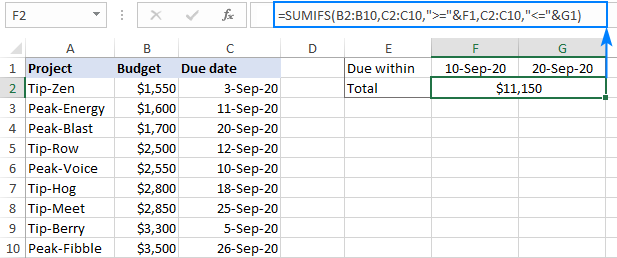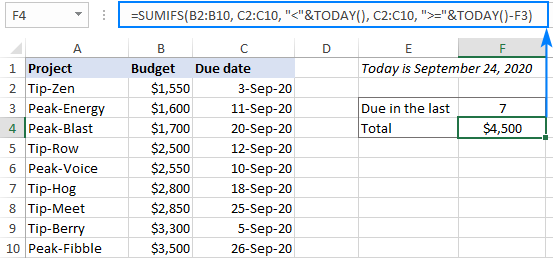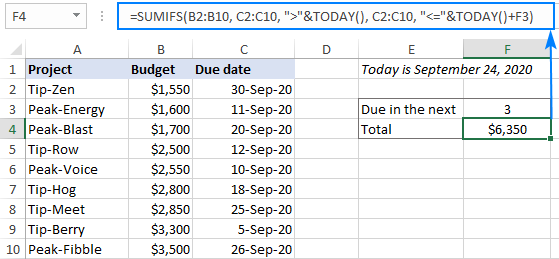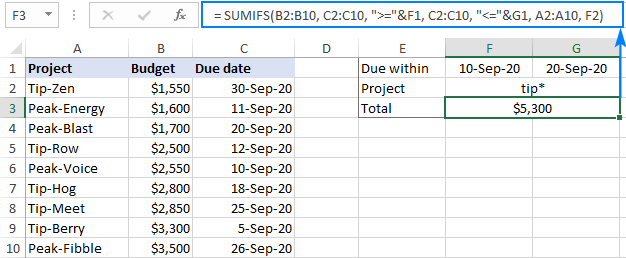# Sum if between two dates in Excel: SUMIFS with date range as criteria

Working on a report, investment plan or any other dataset with dates, you may often need to sum numbers within a specific period. This tutorial will teach you a quick and easy solution - SUMIFS formula with date range as criteria.

On our blog and other Excel forums, people often ask how to use SUMIF for date range. The point is that to sum between two dates, you need to define both dates while the Excel SUMIF function only allows one condition. Luckily, we also have the SUMIFS function that supports multiple criteria.

## How to sum if between two dates in Excel

To sum values within a certain date range, use a SUMIFS formula with start and end dates as criteria. The syntax of the SUMIFS function requires that you first specify the values to add up (sum_range), and then provide range/criteria pairs. In our case, the range (a list of dates) will be the same for both criteria.

Considering the above, the generic formulas to sum values between two dates take this form:

Including the threshold dates:

SUMIFS(sum_range, dates,">=start_date", dates, "<=end_date")

Excluding the threshold dates:

SUMIFS(sum_range, dates,">start_date", dates, "<end_date")

As you can see, the difference is only in the logical operators. In the first formula, we use greater than or equal to (>=) and less than or equal to (<=) to include the threshold dates in the result. The second formula checks if a date is greater than (>) or less than (<), leaving out the start and end dates.

In the table below, suppose you want to sum projects that are due in a particular date range, inclusive. To have it done, use this formula:

`=SUMIFS(B2:B10, C2:C10, ">=9/10/2020", C2:C10, "<=9/20/2020")`

If you'd rather not hardcode a date range in the formula, then you can type the start date in F1, the end date in G1, concatenate the logical operators and cell references and enclose the whole criteria in quotation marks like this:

`=SUMIFS(B2:B10, C2:C10, ">="&F1, C2:C10, "<="&G1)`To avoid possible mistakes, you can supply dates with the help of the DATE function:

`=SUMIFS(B2:B10, C2:C10, ">="&DATE(2020,9,10), C2:C10, "<="&DATE(2020,9,20))`

## Sum within a dynamic range based on today's date

In situation when you need to sum data within a dynamic date range (X days back from today or Y days forward), construct the criteria by using the TODAY function, which will get the current date and update it automatically.

For example, to sum budgets that are due in the last 7 days including todays' date, the formula is:

`=SUMIFS(B2:B10, C2:C10, "<="&TODAY(), C2:C10, ">"&TODAY()-7)`

If you'd rather not include the current date in the final result, use the less than operator (<) for the first criteria to exclude today's date and greater than or equal to (>=) for the second criteria to include the date which is 7 days before today:

`=SUMIFS(B2:B10, C2:C10, "<"&TODAY(), C2:C10, ">="&TODAY()-7)`In a similar manner, you can sum values if a date is a given number of days forward.

For example, to get a total of budgets that are due in the next 3 days, use one of the following formulas:

Today's date is included in the result:

`=SUMIFS(B2:B10, C2:C10, ">="&TODAY(), C2:C10, "<"&TODAY()+3)`

Today's date is not included in the result:

`=SUMIFS(B2:B10, C2:C10, ">"&TODAY(), C2:C10, "<="&TODAY()+3)`## Sum if between two dates and another criteria

To sum values within a date range that meet some other condition in a different column, simply add one more range/criteria pair to your SUMIFS formula.

For example, to sum budgets within a certain date range for all the projects that contain "tip" in their names, extend the formula with a wildcard criteria:

`=SUMIFS(B2:B10, C2:C10, ">="&F1, C2:C10, "<="&G1, A2:A10, "tip*")`

Where A2:A10 are the project names, B2:B10 are the numbers to sum, C2:C10 are the dates to check, F1 is the start date and G1 is the end date.

Of course, nothing prevents you from entering the third criteria in a separate cell too, and referencing that cell like shown in the screenshot:## SUMIFS date criteria syntax

When it comes to using dates as criteria for Excel SUMIF and SUMIFS functions, you wouldn't be the first person to get confused :)

Upon a closer look, however, all the variety of use cases boils down to a few simple rules:

If you put dates directly in the criteria arguments, then type a logical operator (>, <, =, <>) right before the date and enclose the whole criteria in quotes. For example:

`=SUMIFS(B2:B10, C2:C10, ">=9/10/2020", C2:C10, "<=9/20/2020")`

When a date is input in a predefined cell, provide criteria in the form of a text string: enclose a logical operator in quotation marks to start a string and use an ampersand (&) to concatenate and finish the string off. For instance:

`=SUMIFS(B2:B10, C2:C10, ">="&F1, C2:C10, "<="&G1)`

When a date is driven by another function such as DATE or TODAY(), concatenate a comparison operator and a function. For example:

`=SUMIFS(B2:B10, C2:C10, ">="&DATE(2020,9,10), C2:C10, "<="&TODAY())`

## Excel SUMIFS between dates not working

In case your formula is not working or producing wrong results, the following troubleshooting tips may shed light on why it fails and help you fix the issue.

### Check the format of dates and numbers

If a seemingly correct SUMIFS formula returns nothing but zero, the first thing to check is that your dates are really dates, and not text strings that only look like dates. Next, make certain you are summing numbers, and not numbers stored as text. The following tutorials will help you spot and fix these issues.

### Use the correct syntax for criteria

When checking dates using SUMIFS, a date should be put inside the quotation marks like ">=9/10/2020"; cell references and functions should be placed outside the quotes like "<="&G1 or "<="&TODAY(). For full details, please see date criteria syntax.

### Verify the formula's logic

A small typo in a budget could cost millions. A little mistake in a formula might cost hours of debugging time. So, when summing between 2 dates, check if the start date is preceded by the greater than (>) or greater than or equal to (>=) operator and the end date is prefixed by less than (<) or less than or equal to (<=).

### Make sure all ranges are the same size

For the SUMIFS function to work correctly, the sum range and criteria ranges should be equally sized, otherwise a #VALUE! error occurs. To fix it, ensure that all criteria_range arguments have the same number of rows and columns as sum_range.

That's how to use the Excel SUMIFS function to sum data in a date range. If you have some other interesting solutions in mind, I'll be really grateful if you share in comments. Thank you for reading and hope to see you on our blog next week!